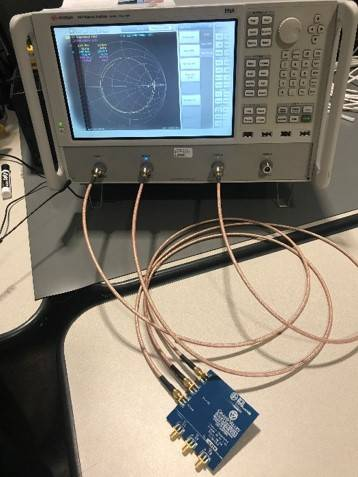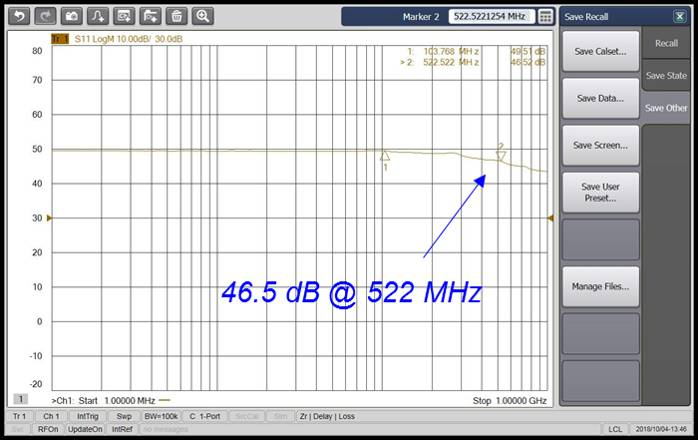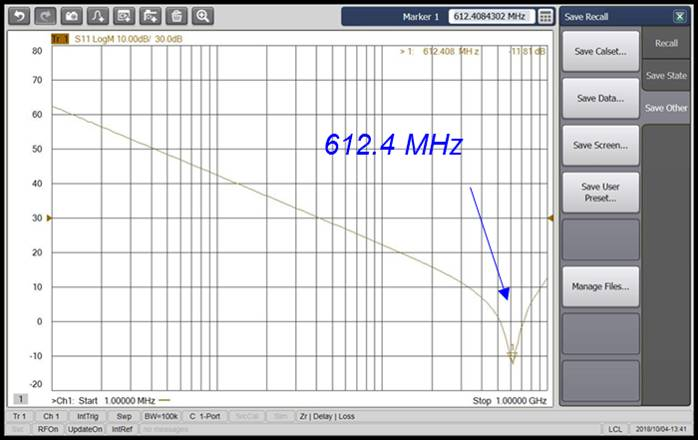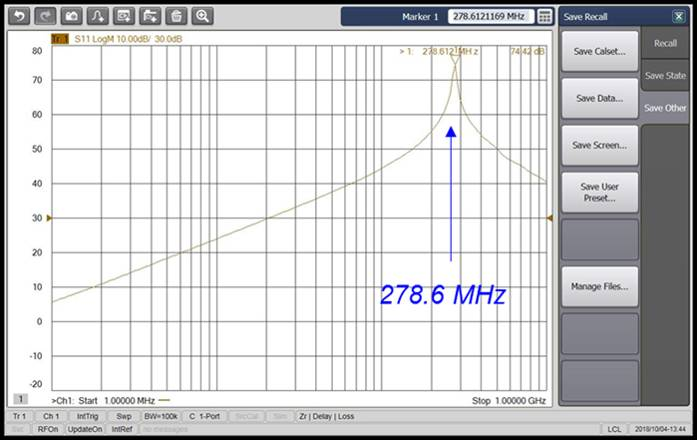# Thursday Afternoon Session II (3:00-4:30 pm)

## Non-Ideal Behavior of Resistors

• Ideal vs. non-ideal model of a resistor, impedance curves
• Effect of the parasitics, self-resonant frequency
• Effect of the connecting trace length on impedance
• Measurement – Resistor impedance and PCB trace impact

## Non-Ideal Behavior of Capacitors

• Ideal vs. non-ideal model of a capacitor, impedance curves
• Effect of the parasitics, self-resonant frequency
• Effect of the connecting trace length on impedance
• Measurement – Capacitor impedance and PCB trace impact

## Non-Ideal Behavior of Inductors and PCB Traces

• Ideal vs. non-ideal model of an inductor and a PCB trace, impedance curves
• Effect of the parasitics, self-resonant frequency
• Effect of the connecting trace length on impedance
• Measurement – Inductor impedance and PCB trace impedance